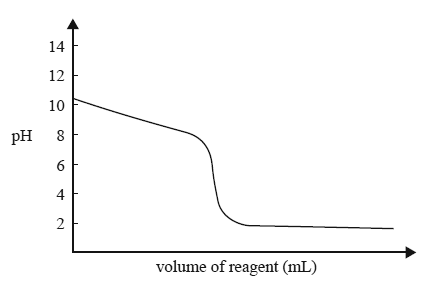Volumetric analysis (2014 VCE) 1) The diagram below represents the titration curve for the reaction between a particular acid and a particular base.The equation that best represents the reaction described by the titration curve is A. HCl(aq) + NH3(aq)  NH4Cl(aq) B. HCl(aq) + NaOH(aq)  NaCl(aq) + H2O(l) C. CH3COOH(aq) + NH3(aq)  CH3COONH4(aq) D. CH3COOH(aq) + NaOH(aq)  CH33OONa(aq) + H2O(l) Solution2) Oil absorbs a small amount of water from its surroundings. In 1935, Karl Fischer, a German chemist, published a technique for the determination of the water content in oil samples. This technique also involves the conversion of sulfur dioxide to sulfate. In this analytical technique, the reactants – iodine, sulfur dioxide and a base – are all dissolved in methanol. The base is an organic compound and is represented by B in the balanced equation for this reaction. States are not included in this equation. H2O + I2 + SO2 + CH3OH + 3B => [BH]SO4CH3 + 2[BH]I i. What is the mole ratio between iodine and water in this reaction? Solutionii. The iodine titrating agent was prepared by dissolving 15.0 g of iodine, I2, in methanol using a volumetric flask and making up the volume to 500.0 mL. A 10.0 mL sample of oil was analysed using the iodine solution. The mean titre was found to be 4.95 mL. M(I2) = 253.8 g mol–1 M(H2O) = 18.0 g mol–1 Determine the mass of water present in a 10.0 mL sample of oil. Solutioniii. Determine the percentage by mass of water present if the density of the oil sample is 0.918 g mL–1. Solution